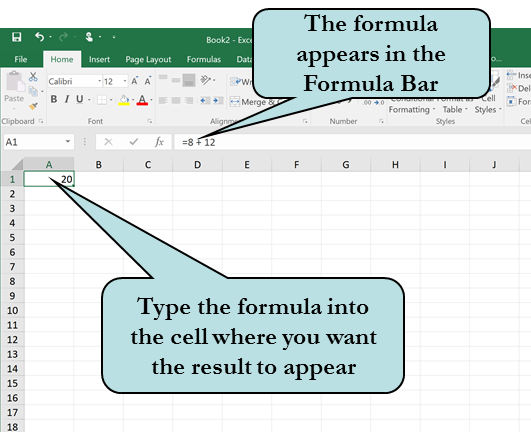# A Look at Excel Formulas and the Order of Operations

Posted by Roger Hyttinen on

## Happy Independence Day to all of my American friends!

Today, I have a beginners tip for you, in which we take a look at formulas and the order of operations in Excel. Formulas perform calculations such as addition, subtraction, multiplication and division on your spreadsheet. You type the formula in the cell where you wish the result to appear. The formula itself does not appear in the cell rather the result of the formula. The formula appears in the formula bar.To tell Excel that you are about to enter a formula, you must precede it with an equal (=) sign. For instance, if you wished to find the total for the numbers 8 and 12, we would enter:

=8+12 in the active cell.

Valid mathematical operators that you can use in your formulas include:

- Subtraction
* Multiplication
/ Division

In addition to performing calculations on actual numbers (= 11 + 2 + 5), you can perform calculations on the value of cells by using Cell References in your formula. If you wanted to find to total of the cells A3, B6 and C7, you would enter the formula:

=A3 + B6 + C7

Excel calculates by hierarchy and order of operations. When Excel performs a calculation it does so in the following order:

1. Exponentiation
2. Multiplication and Division

= A2 + B3 * B7

The B3 * B7 part of the formula is calculated first, then the result is added to A2.

If a formula contained both a multiplication and a division operator Excel would calculate them from left to right. The same applies for subtraction and addition.

We can change the order in which Excel performs its calculations by enclosing the relative function in parenthesis. Let's say we had the formula =5 + 10 * 5 the result would be 55. If we changed this to: =(5 + 10) * 5 the result would be 75.

## To Create a Simple Formula

1. Click in the cell where you want the result of your formula to display.
2. Type: =
3. Type the formula using hard-coded numbers or cell references.
4. Press Enter to confirm the formula.
Our Excel 2016: Up To Speed guide is now available in printable PDF format from this site. The Up To Speed series is designed to help you get productive quickly with your software. Check back as new titles will be added regularly.  Also available as an ebook from Amazon, iTunes Books and Kobo.